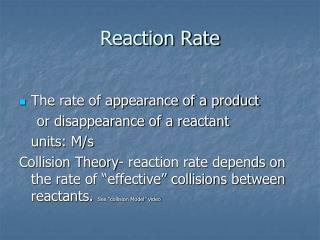# Reaction Rate - PowerPoint PPT PresentationDownload PresentationReaction Rate

Reaction RateDownload Presentation## Reaction Rate

- - - - - - - - - - - - - - - - - - - - - - - - - - - E N D - - - - - - - - - - - - - - - - - - - - - - - - - - -
##### Presentation Transcript

1. Reaction Rate • The rate of appearance of a product or disappearance of a reactant units: M/s Collision Theory- reaction rate depends on the rate of “effective” collisions between reactants. See “collision Model” video

2. Factors that affect reaction rate • Nature of reactants • Ionic reactions tend to be fast (double displacement) • Molecular reactions are slower (bonds rearrange) • Concentration • Rate = k[A]m[B]n (rate constant x product of concentration of reactants) • Increase pressure on a gas • Temperature • Directly proportional • Surface area • Catalyst present

3. activated complex- a transitional structure created from an effective collision. • activation energy- the energy required to form the activated complex

4. Ef = activation energy for forward reaction = 30kJ • Er = activation energy for the reverse reaction = 60kJ

5. catalyst a substance that speeds up a chemical reaction without being changed itself. It lowers the activation energy needed for a reaction to occur.

6. Exothermic- gives off heat • Products have less energy than reactants • Tend to be spontaneous • Endothermic- absorbs heat • Products have more energy than reactants

7. N2(g) + 3H2(g)  2NH3(g) If the rate of disappearance of H2 is .048 M/s, what would be the rate of formation of ammonia? .048M/s x 2 mol NH3 = .032 M/s 3 mol H2 Use stoichiometry to relate the rate of one species to the rate of another species in the same reaction (under the same conditions)

8. Predict • Draw a graph of concentration vs. time for A + 2B  3C http://www.chm.davidson.edu/vce/kinetics/ReactionRates.html legend ----- [A] …….[B] ___ [C] Concentration (M) Time

9. Reaction rate varies directly as the product of the concentrations of the reactants in the rate determining step. Rate Law Rate = k[A]n[B]m K is the rate constant, increases with temp. [A], [B] are molar concentrations of reactants n, m – reaction order, determined experimentally Overall reaction order = n + m

10. 0 order: Rate = k 1st order: Rate = k [A] If [A] doubles, rate doubles If [A] triples, rate triples 2nd order: rate = k [A]2 If [A] doubles, rate quadruples (22) If [A] triples, rate is 9x (32) 3rd order: rate = k [A]3 If [A] doubles, rate is 8x (23) If [A] triples, rate is 27x (33)

11. A + B g C Determine the rate expression for the above reaction. Rate = k[A]2 Determine the rate constant, k. K = .0076/(.1)2 = .76 M-1s-1

12. C2H4Br2(l) + 3I-(aq) g C2H4(l) + 2Br-(aq) + I3-(aq) • Three particles colliding with another is unlikely to occur in one step. • Actual reaction mechanism: C2H4Br2 + I- g C2H4Br- + IBr (slow) C2H4Br- g C2H4 + Br- (fast) IBr + I-g Br- + I2 (fast) I2 + I-g I3- (fast) Rate = k[C2H4Br2][I-] overall order of a reaction = two

13. Reaction Mechanisms NO2 + F2gNO2F + F slow F + NO2g NO2F fast 2NO2 + F2g2NO2F overall • The rate law should have the reactants from the slow (rate determining) step in it. • Rate = k [NO2][F2] • Catalyst- appears as a reactant and a product • Intermediate- is created then used up (not a reactant or product.

14. You can determine the rate law from a balanced equation IF it is an elementary reaction (one step) or if you know the slow step. Write the rate law for the single elementary reaction below. H2(g) + Br2(g)  2HBr(g) Rate = k [H2][Br2]

15. Half life • T1/2 is the time required for the concentration of a reactant to drop by one half. • The longer the half life, the more stable the compound.

16. N2O5(sol) 2NO2(sol) + ½O2(g) What is the half life in this reaction? 2.5 hours What concentration of N2O5 will be left after two half lives (5 hour)? .37M

17. Summary

18. Based on these graphs of concentration of hydrogen peroxide vs. time, what order reaction is this? Which graph is most linear?

19. Activation energy • k = Ae(-Ea/RT) Arrhenius equation • A is a constant related to the number, orientation and frequency of collisions occurring between the particles in the reaction, called frequency factor • k is rate constant • R is universal gas constant • T is absolute temperature • Ea is activation energy (J/mol) • e is 2.71828 . . . As activation energy increases, reaction rate (and k) decreases- a smaller fraction of the molecules will have enough energy to react

20. As seen on the AP Chem equations sheet Y = mx + b A graph of lnk vs 1/T will be a line with slope –Ea /R and a y-intercept of lnA.

21. Sample problem From this data, calculate the activation energy.

22. Slope = -6 – (-10.4) .00195-.00215 = -1.9 x 104 Slope = - Ea R Ea =-(slope)(R) = -(-1.9x104 K)(8.31J/mol∙K)(1kJ/1000J) =1.6 x 102 kJ/mol